# Fractional Order PID Design for MPPT-Pitch Angle Control of Wind Turbine Using Bat Algorithm

Fractional Order PID Design for MPPT-Pitch Angle Control of Wind Turbine Using Bat Algorithm
Laboratory of Power Systems Control and Electrical Networks (LACoSERE), University of Amar Thelidji, Laghouat, Alegria

Corresponding Author Email:
ou.maroufi@lagh-univ.dz
Page:
35-42
|
DOI:
https://doi.org/10.18280/ama_a.562-402
23 May 2019
|
Accepted:
15 August 2019
|
Published:
31 December 2019
| Citation

OPEN ACCESS

Abstract:

Extract the maximum power and protection of the Wind Turbine (WT) become critical issues for the development of the renewable energy process. For this purpose, a competitive Fractional Order PID (FOPID) controller adjusted with Bat Algorithm (BA) optimisation technique is proposed for the control of WT based Permanent Synchronous Generator WT-PMSG. The principals objectives of control are tracking the Maximum Power Point (MPPT) in low wind speed region and protect the WT   in high wind speed region using Pitch Angle control. Performance of proposed optimal FOPID design is compared with the classical PI controller under low-high wind speed profiles. Simulation results improve the effeteness and the superiority of FOPID over PI controller in terms; response, overshoots and indices performance

Keywords:

wind turbine, PMSG, MPPT, pitch angle, FOPID controller

1. Introduction

In the last decades, wind energy (WE) conversion system gained more attention because of both the development of technological innovation and the reduction costs of WE systems. In this context the variable pitch wind turbine driven by the permanent synchronous generator is one of the promising ones due to its advantages; no gearbox, high power and low cost. [1-2].

The variable wind speed characterized by two principals controls; in the low-speed region, it must be keeping the maximum power for each value of wind speed via MPPT control. When the wind speed above the rated value, it should operate the WT at the nominal power (or generator speed) by run the pitch angle control .

In this context, the linear PI controller is largely used in the literature . However, the performance of the conventional controller is limited over the nonlinearity of WT and the high disturbances of wind speed profiles. Consequently, many researchers try to enhance the results by using new strategies to overcome the deficits of the classical controller. We can cite some of these works; As outlined in , Second Order Sliding Mode (SOSM) controller is adopted in low-speed case.  From the results, we observe the effeteness of HOSM controller by removing the chattering problem. Takagi-Sugeno (T-S) fuzzy controller is also employed in  for the objective of MPPT control. Performance of T-S fuzzy is tested under a wide variety of wind speed. The results prove the robustness of T-S compared than the PI controller. Fuzzy Sliding (FS) mode design is presented in reference . The purpose of this hybridization is to benefits the performances of fuzzy logic and sliding mode controllers.

Combined MPPT-Pitch angle control using Neural Networks (NN) introduced in . From the results, we can note the high performance of this technique. However, the main problem of the NN controller is the large time of learning.

Recently, a new extension of conventional PID controller has been popularly investigated called Fractional Order PID controller. The orders of the integrator and the derivative can be fractional values. Where this property gives more freedom than the PID controller [9-10].

Owing to the complexity of the studied system, the direct synthesis of controllers parameters is not straightforward. Therefore, different optimization techniques are used to find the best controller's parameters. Some of these optimizations techniques are used in engineering systems are; Genetic Algorithm (GA) , Particle Swarm (PS) , Gray wolf , chaotic algorithm . Bat Algorithm optimization method is one of the most widely used methods for solving optimization problems. BA is inspired by the echolocation behaviour of bats to detect the prey and backgrounds .

In this paper, BA optimization technique is introduced to find the best parameters of FOPID controller for solving the MPPT- pitch angle problems of WT-PMSG system. The performance and the robustness of the proposed design is compared with the classical PI controller, under wide fluctuations of wind speed profiles in low-high regions.

The remainder of this paper is organized as follows. Both of modelling of WT-PMSG and the control of the objective are explained in Section 2. The proposed FOPID controller and the BAT algorithm optimisation technique are presented in Section 3. Then, the simulation results and their discussions are drawn in Section 4. Finally, the main conclusions are presented in Section 5.

2. Modelling of WT-PMSG

The global WT-PMSG system is presented in Figure 1, which composed by variable pitch wind turbine coupled with PMSG. In this part, the captured wind energy is transformed into electrical energy. Then the variable nature of wind speed, obligate to in install DC/DC rectifier to maintain the voltage in one level. Finally, the DC/AC rectifier is added to assure the frequency value equal with the grid.  In this work, we concentrate on control of the generator side mentioned in the overall system.

## 1.png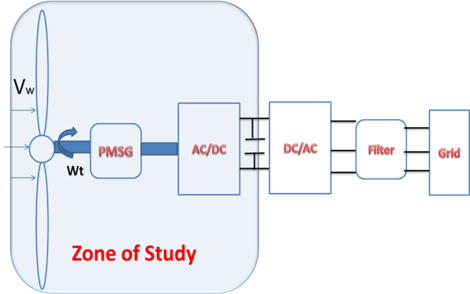Figure 1. Global WT system

According to the Betz theory, the mechanical power   captured by the WT from each wind speed value , be expressed by:

$P_{t}=\frac{1}{2} \rho \pi R^{2} v_{w}^{3} C_{P}(\lambda, \beta)$            (1)

where, $C_{P}$ is the power coefficient, $\omega_{t}$ is the mechanical rotation speed, $\lambda$ is the tip speed ratio, $v_{w}$ is the wind speed, $\rho$ is the air density and $R$ is the blade length. The tip speed ratio is defined as:

$\lambda=\frac{R \omega}{v_{w}}$     (2)

where $\omega$ is the generator speed

$C_{P}=0.5176\left(\frac{116}{l_{i}}-0.4 \beta-5\right) e^{-\frac{21}{l_{i}}}+0.0068 \lambda$      (3)

where

$\frac{1}{l_{i}}=\frac{1}{\lambda+0.08 \beta}-\frac{0.035}{\beta^{3}+1}$          (4)

2.1 WT operating regions

The typical power curve of a WT [15-16] is presented in Figure 2. From this Figure, we can see three different operational regions. The first is the cut-speed region, wherein this situation; the turbine should be stopped and disconnected from the grid. Also, the second region called the low-speed region that is bounded by the $V_{\text {cut}}$  and the rated speed $V_{\text {rated}}$, in this case, the turbine produces the maximum power and by maintaining the tip speed ratio at the optimal value and the efficiency of power at maximum value. Besides, in this region, the pitch angle is deactivated. Finally, as the wind speed attained the $V_{c u t-o u t}$, the control unit moved to the third region. Which, in this region, the WT control unit tunes the pitch angle to keep the rated output power value.

## 2.pngFigure 2. The ideal power curve of a wind turbine

2.2 MPPT control

As illustrated in Figure 3, in the low-speed case, it should be captured the maximum power for each wind speed value. This reached by maintained the power coefficient at its maximum value $C_{pmax }=0.48,$ by varying the rotor speed to keep the $\lambda$ equal to the optimal value $\lambda_{\text {opty}}=8.1,$ which is

$\omega_{o p t}=\frac{\lambda_{o p t} v_{w}}{R}$      (5)

## 3.pngFigure 3. Power coefficient

2.3 Pitch angle control

In the case of high wind region, it is necessary to limit the rotational generator speed to avoid the damage of the turbine and in other words, the output power of WT can be regulated by pitch angle control see Figure 4. The control strategy implemented is as follows:

$\left\{\begin{array}{c}\beta_{r e f}=\beta_{0}=0, \text { for } P_{g} \leq P_{\text {rated}} \\ \beta_{\text {ref}}=\frac{\Delta \beta}{\Delta P}\left(P_{g}-P_{\text {rated}}\right), \text {for } P_{g}>P_{\text {rated}}\end{array}\right.$        (6)

with $\beta, P_{q}, P_{\text {rated}}$ are respectively pitch angle, generated power and rated power.

## 4.pngFigure 4. Characteristic of the WECS for different pitches

2.4 Modeling of the PMSG

The electrical and mechanical equations of PMSG model are given by:

$\left\{\begin{array}{c}v_{d}=R_{s} i_{d}+L_{d} \frac{d i_{d}}{d t}-L_{q} \omega i_{q} \\ v_{q}=R_{s} i_{q}+L_{d} \frac{d i_{q}}{d_{t}}-\omega\left(L_{d} i_{d}+\psi\right)\end{array}\right.$        (7)

where $\left(v_{d}, v_{q}\right)$ are the stator voltages in the d-q axis, $\left(i_{d}, i_{q}\right)$ are the currents in the d-q axis, $\omega=p \omega_{t}$ is the electrical rotation speed, $R_{s}, L_{d}$ and $i_{q}$ respectively, are the stator resistance, the direct and the quadrature inductance, $\psi$ represents the flux linkage of permanent magnets and is $p$ the number of poles, The smooth-air-gap of the synchronous machine is considered, $L_{d}=L_{q}=L$ The electromagnetic torque in the d-q reference frame is given by :

$\Gamma_{e}=\frac{3}{2} p\left(\left(L_{d}-L_{q}\right) i_{d} i_{q}+\psi i_{q}\right)=\frac{3}{2} p \psi i_{q}$         (8)

## 5.png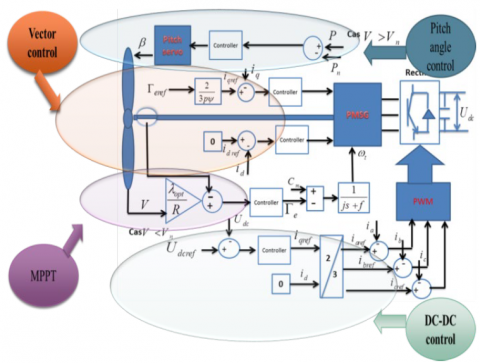Figure 5. Global objectives control

The control of the generator side based to set the d-axis reference current equal to zero $\left(i_{d r e f}=0\right)$ for reduced the resistive losses in the stator windings. In another hand, the quadrature reference current $\left(i_{q r e f}\right)$ is proportional to the torque reference ($\Gamma_{e r e f}$) that is generated from an MPPT controller and varies under changes of wind velocities. Further, the rectifier controlled using a hysteresis-band current control technique, where the three-phase line currents are compared to the three-phase reference currents applied to a hysteresis controller for generating pulse-width modulation (PWM) pulses. Ultimately, Figure 5 presents objectives control of the studied system .

3. Controllers Designs

3.1 Fractional order PID controller

A new design of linear PID controller named Fractional Order PID $\left(F O P I D \text { or } P I^{\lambda} D^{\mu}\right)$ controller proposed by Podlubny [18-19]. which can be involving both the integrator and the differentiator in order $\lambda$ and $\mu,$ respectively. Consequently, As shown in Figure 6 the FOPID strategy has more degrees of freedom than the conventional controller [20-21]. Fractional operators can be derived by various definitions. However, the most frequently used are Riemann-Liouville definition given by Eq. (8)

## 6.pngFigure 6. Plan representation of fractional $P I^{\lambda} D^{\mu}$ and classical controllers

$_{0}^{\alpha} D f(t)=\frac{1}{\Gamma(m-\alpha)} \int_{0}^{t} \frac{f^{m}(\tau)}{(1-\tau)^{\alpha-m+1}}$       (9)

To implement the fractional order controller both the numerical simulations and the industrial process, this needs a method of approximation with integer-order transfer functions. Generally, various approximations are introduced in this procedure, such as Crone, Carlson, the high frequency continued fraction and the low frequency continued fraction. In this study, the Crone approximation is adopted, where this approximation uses a recursive distribution of N poles and N zeros.

$C(s)=k^{\prime} \prod_{n=1}^{N} \frac{\left(1+\omega_{z n}\right)}{\left(1+\omega_{p n}\right)}$         (10)

where $k^{\prime}$ is an adjusted static gain. Zeros and poles are determined inside a frequency range and are given below:

$\alpha=\left(\frac{\omega_{h}}{\omega_{l}}\right)^{\frac{v}{n}}$     (11)

$\eta=\left(\frac{\omega_{h}}{\omega_{l}}\right)^{1-v / n}$           (12)

$\omega_{z l}=\omega_{l} \sqrt{\eta}$       (13)

$\omega_{p n}=\omega_{z, n-1} \alpha, \quad n=1 \ldots N$         (14)

$\omega_{Z n}=\omega_{p, n-1}, \quad n=1 \ldots N$            (15)

In this paper, the frequency range is selected as $\omega_{l}=0.001$ $\operatorname{rad} / \mathrm{s} \operatorname{rad} / \mathrm{s}, \omega_{h}=10 \mathrm{rad} / \mathrm{s}$ and the number of zeros and

poles is taken $N=3 .$ The mathematical expression of fractional order PID (or $\mathrm{PI}^{\lambda} \mathrm{D}^{\mu}$ ) controller can be presented by the following differential equation:

$u(t)=k_{p} e(t)+k_{i} D_{t}^{-\lambda} e(t)+k_{d} D_{t}^{\mu} e(t)$   (16)

where $u(t)$ is the control signal and $e(t)$ is the error, $k_{p}$ is the proportional gain, $k_{i}$ is the integrator gain and $k_{d}$ is the derivative gain.

3.2 Optimization problem

In our study, the  selected  fitness function is  the Integral of the Absolute  Error (ISE) criterion as a performance index and is given below

$J=I A E=\int_{0}^{t_{s i m}}|e(t)| d(t)$        (17)

The optimization problem can be formulated as follows:

Minimize:

$J\left(k_{p}, k_{i}\right)\left\{\begin{array}{l}k_{p}^{\min }<k_{p}<k_{p}^{\max } \\ k_{i}^{\min }<k_{i}<k_{i}^{\max }\end{array}\right.$      (18)

$J\left(k_{p}, k_{i,} k_{d}, \lambda, \eta\right)\left\{\begin{array}{c}k_{p}^{\min }<k_{p}<k_{p}^{\max } \\ k_{i}^{\min }<k_{i}<k_{i}^{\max } \\ k_{d}^{\min }<k_{d}<k_{d}^{\max } \\ \lambda^{\min }<\lambda<\lambda^{\max } \\ \eta^{\min }<\eta<\eta^{\max }\end{array}\right.$        (19)

3.3 Bat algorithm

The BA is a new swarm intelligence method proposed by Yang, inspired by the echolocation phenomenon in bats locate the prey and backgrounds [22-23]. Normally, the reflected sound pulses by barriers are transformed to the frequency and in case of prey is nearby, bat increase the emits pulses rate and regulate the frequency, which increases the precision search. Yang proposed these general rules :

Bats utilize echolocation method to sense distance and they know the difference between background barriers/prey with some magical manner;

Bats fly with stochastic manner, with these proprieties: velocity $\mathrm{v}_{\mathrm{j}}$ at position $\mathrm{x}_{\mathrm{j}},$ constant frequency $f_{\text {min }},$ varying loudness $A_{0}$ and wavelength $\lambda$ in purpose to manhunt prey. They can automatically tune the frequency of their emitted pulses, in additional adjust the rate of pulse emission in range $\mathrm{r} \in[0,1],$ depending on the proximity of their aim;

While the loudness can be changed in many ways, we assume that the loudness changes from large (positive) $A_{0}$ to the lowest constant value $\mathrm{A}_{\mathrm{min}}$;

for each bat ( $j$ ) has these proprieties; velocity $v_{j}$ and position $\mathrm{x}_{\mathrm{j}}$ in a d-dimensional search space, the novel solutions velocities $v_{\mathrm{j}}^{t}$ and $x_{\mathrm{j}}^{t}$ at time step $t$ can be written as follows:

$f_{\mathrm{j}}=f_{\min }+\left(f_{\max }-f_{\min }\right) \alpha$             (20)

$v_{\mathrm{j}}^{t}=v_{\mathrm{j}}^{t-1}+\left(x_{\mathrm{j}}^{t-1}-x^{*}\right) f_{\mathrm{j}}$         (21)

$x_{j}^{t}=x_{j}^{t-1}+v_{j}^{t}$      (22)

where, $\alpha$ is a random value in range [0 1] written by uniform distribution also $x^{*}$ presents the available global best location. In local search, one the best solution is chosen on all solutions among $n$ bats. As the product $f_{\mathrm{j}}\left(\text { or } \lambda_{\mathrm{j}}\right)$ is the speed increment. For implementation, any bat is stochastically assigned a frequency where is written from $\left(f_{\min }-f_{\max }\right) .$ A new solution for each bat can be generated locally by:

$x_{n e w}=x_{o l d}+\varepsilon A^{t}$           (23)

where, $\varepsilon \in[0,1]$ is a random number, while $A^{t}=<A_{j}^{t}>$ presents the mean loudness of the bats in this time step. In case, the bat detects its prey, the loudness decreases and the rate of pulse emission increase. The loudness is typically selected in range $\left[\mathrm{A}^{0}, A_{\min }\right]=[1,0] .$ Assuming $\mathrm{A}_{\mathrm{min}}=0$ means that a

bat around to the prey and in this case it stops sending any noise. The loudness and pulse emission rate can drown as follow:

$r_{\mathrm{j}}^{t+1}=r_{\mathrm{j}}^{0}[1-\exp (-\gamma t)], A_{\mathrm{j}}^{t+1}=\beta A_{\mathrm{j}}^{t}$       (24)

In most cases can be selected $\beta=\gamma=\left[\begin{array}{ll}0.9 & 0.975\end{array}\right]$. The pseudo-code of BA is summarized in the following:

 Bat Algorithm Identify Objective function $f(x), x=\left(x_{1}, x_{2}, \ldots, x_{10}\right)^{T}$ Initialize the bat population: $x_{\mathrm{i}}(\mathrm{j}=1,2, \ldots, 10)$ and $v_{i}(\text { npop }=20)$ Define pulse frequency : $f_{\mathrm{j}}$ at $x_{\mathrm{j}},$ which $\left(f_{\min }=0, f_{\max }=1\right)$ Initialize pulse rates and the loudness: $(\boldsymbol{r}=\mathbf{0 . 5}, \boldsymbol{A}=\mathbf{0. 5})$ Define the boundaries of the parameter: $\left(\boldsymbol{L}_{\boldsymbol{b}}; \boldsymbol{U}_{\boldsymbol{b}}\right)$; see Table 1 1: while $\left(\boldsymbol{t}<\boldsymbol{t}_{\max }\right) ; \boldsymbol{t}_{\text {max }}:$ Max number of iterations Tuning frequency generate novel solutions updating velocities and locations/solutions [Eqs (20) to (22)], $2: i f(r a n d>r)$ Choose a solution between the best solutions. Generate a local solution around the selected best solution, [Eq(23)] 3: end if Generate a new solution by flying randomly, 4: if $\left(\text { rand } 4. Simulation Results For checking the robustness of the proposed FOPID controller, several simulations are carried out on WT-PMSG system using Matlab/Simulink. The parameters of the WT-PMSG are tabulated in Table 1. The optimization results and the obtained optimal gains of the FOPID and PI controllers are provided in Table 2. Table 1. Wind conversion system parameters  Parameter Value Blade length (R) Air density ($\rho$) stator inductance (L) Friction coefficient (f) Moment of inertia (J) Stator resistance (Rs) Magnetic flux ($\psi$) Number of poles (p) 2[m] 1.225 [$k g \cdot m^{-1}$] 0.174 [mH] 0.005 [$N \cdot m^{-1}$] 0.089 [$k g . m^{2}$] 0.00829 [Ω] 0.071[wb] 6 Table 2. IAE results based BA  Controllers MPPT ISE Pitch Angle ISE PI kp 41.1167 10.3890 87.1653 328.52 ki 90.8445 2.7957 kp 73.5364 49.9984 ki 2.4781 8.5219 0.10118 FOPID kd 91.4274 0.284295 290.0265$\lambda$0.8904 0.986747$\eta$0.9900 0.979805 In the beginning, as shown in Figure 7, the ability of the investigable controllers are verified under variable low wind speed profile, varying among 10-13 [m/s]. Figures$8-9$illustrate waveforms of the tip speed ratio$(T S R$or$\lambda$) and the power coefficient$\left(C_{P}\right) .$From the zoomed figures, it can be seen that the proposed optimal FOPID controller has superiority to track the optimal value of$T S R=8.1$and the maximum power coefficient$C_{P}=0.48$compared than the optimal PI controller ## 7.pngFigure 7. Low-speed profile ## 8.pngFigure 8. Tip speed ## 9.pngFigure 9. Power coefficient Consequently, Figures$9-10$have confirmed that the FOPID controller has fast tracks of the desired generator speed ($\omega_{r e f}$) and the output power$\left(P_{\text {ref}}\right)$references. ## 10.pngFigure 10. Power generator Further, the suggested controller has a low fitness function$\left(I S E_{F O P I D}=8.5219\right) \quad$compared$\quad$to the counterpart$\left(I S E_{F F P I D}=10.3890\right),$see Table$2 .$To further show the robustness of FOPID controller, we have adopted a high wind speed profile, see Figure$11 .$Where the wind speed in infinite time$(t=5)$has attained the rated value$\left(v_{\text {rated}}=\right.$13.94 ), we denote that in this scenario the pitch angle control is activated. Figure 12 found that the FOPID controller has faster and low overshoot under the variation of wind speed than the PI controller, see zoomed Figure$12 .$This note can be also confirmed by Table$2 .$We can observe that the proposed controller has the lowest fitness function$\left(I S E_{F O P I D}=\right.$290.0265 compared to the classical controller$\left(I S E_{P I}=\right.$328.52). ## 11.pngFigure 11. Wind speed profile ## 12.png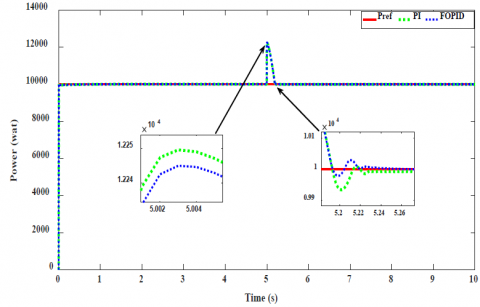Figure 12. Power generator After the verification of good performances obtained with the proposed controller compared than the conventional controller, the rest of the control system is adopted using FOPID controller. Figures 13-14 exhibit the vector control of PMSG, the proposed control strategy has a proper response and it reached the desired references$\left(i d_{r e f}=0, i_{q r e f}=\frac{2}{3 p \psi}\right)$despite the changes in wind speed. ## 13.png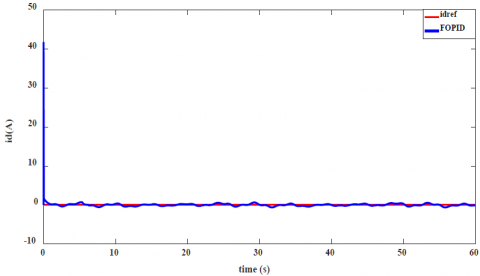Figure 13. d-axis current ## 14.png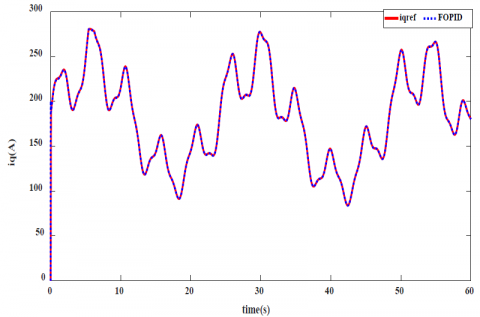Figure 14. q-axis current Finally, the bus voltage control is illustrated in Figure 15, the result found that the proposed scheme achieves and ensures the DC voltage at the reference despite the disturbances. ## 15.png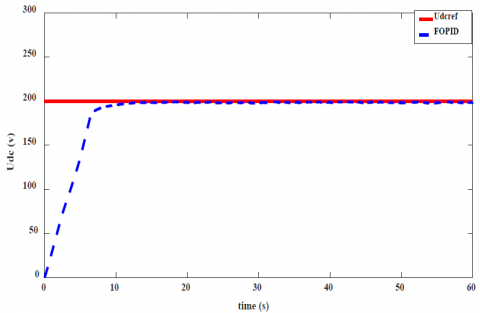Figure 15. Bus voltage tracking 5. Conclusion In this work, effective control of variable wind speed turbine coupled with PMSG, based Fractional Order PID controller strategy tuned with Bat Algorithm optimization method. The control objectives are; tracking the maximum power (MPPT) in the low-speed region case. Furthermore, control the pitch angle, when the wind speed attends the rated value for limiting the output power, avoiding the destruction of WT and also ensuring the continuity of service. Then, the performance of the proposed controller is compared with the optimal PI controller under low-high wind speed profiles. Simulation results prove that the optimal FOPID controller has more performance than the classical controller in terms; response, robustness under the changes of wind speed and low error. Eventually, we can be summarized that the WT-PMSG have effectively controlled by optimal FOPID controller strategy. References  Munteanu, I. (2008). Wind turbine control systems. Principles, modelling and gain scheduling design. Fernando D. Bianchi, Hernán De Battista and Ricardo J. Mantz, Springer, London, 2006. No. of pages: XIX+ 207. Price:$119. International Journal of Robust and Nonlinear Control: IFAC‐Affiliated Journal, 18(7): 796-797. https://doi.org/10.1002/rnc.1263

 Xu, F., Zhang, J., Cheng, M. (2011). Analysis of double objectives controls for wind power generation system with frequency separation. In 2011 4th International Conference on Electric Utility Deregulation and Restructuring and Power Technologies (DRPT), 1366-1371. https://doi.org/10.1109/DRPT.2011.5994109

 Lalouni, S., Rekioua, D., Idjdarene, K., Tounzi, A. (2015). Maximum power point tracking based hybrid hill-climb search method applied to wind energy conversion system. Electric Power Components and Systems, 43(8-10): 1028-1038. https://doi.org/10.1080/15325008.2014.999143

 Podlubny, I. (1999). Fractional-order systems and PI/sup/spl lambda//D/sup/spl mu//-controllers. IEEE Transactions on automatic control, 44(1): 208-214. https://doi.org/10.1109/9.739144

 Dulău, M., Gligor, A., Dulău, T.M. (2017). Fractional order controllers versus integer order controllers. Procedia Engineering, 181: 538-545. https://doi.org/10.1016/j.proeng.2017.02.431

 Ounnas, D., Ramdani, M., Chenikher, S., Bouktir, T. (2015). A fuzzy tracking control design strategy for wind energy conversion system. In 2015 International Conference on Renewable Energy Research and Applications (ICRERA), 777-782. https://doi.org/10.1109/ICRERA.2015.7418517

 Kahla, S., Soufi, Y., Sedraoui, M., Bechouat, M. (2017). Maximum power point tracking of wind energy conversion system using multi-objective grey wolf optimization of fuzzy-sliding mode controller. International Journal of Renewable Energy Research (IJRER), 7(2): 926-936.

 Dahbi, A., Nait-Said, N., Nait-Said, M.S. (2016). A novel combined MPPT-pitch angle control for wide range variable speed wind turbine based on neural network. International Journal of Hydrogen Energy, 41(22): 9427-9442. https://doi.org/10.1016/j.ijhydene.2016.03.105

 Zamani, M., Karimi-Ghartemani, M., Sadati, N., Parniani, M. (2009). Design of a fractional order PID controller for an AVR using particle swarm optimization. Control Engineering Practice, 17(12): 1380-1387. https://doi.org/10.1016/j.conengprac.2009.07.005

 Yang, B., Yu, T., Shu, H., Han, Y., Cao, P., Jiang, L. (2019). Adaptive fractional‐order PID control of PMSG‐based wind energy conversion system for MPPT using linear observers. International Transactions on Electrical Energy Systems, 29(1): e2697. https://doi.org/10.1002/etep.2697

 Oztürk, A., Tosun, S., Alkan, S., Avci, E., Bilir, R. (2015). Speed control of wind turbine rotor using genetic algorithm. In 2015 9th International Conference on Electrical and Electronics Engineering (ELECO), 822-826. https://doi.org/10.1109/ELECO.2015.7394491

 Kahla, S., Soufi, Y., Sedraoui, M., Bechouat, M. (2015). On-Off control based particle swarm optimization for maximum power point tracking of wind turbine equipped by DFIG connected to the grid with energy storage. International Journal of Hydrogen Energy, 40(39): 13749-13758. https://doi.org/10.1016/j.ijhydene.2015.05.007

 Asgharnia, A., Shahnazi, R., Jamali, A. (2018). Performance and robustness of optimal fractional fuzzy PID controllers for pitch control of a wind turbine using chaotic optimization algorithms. ISA transactions, 79: 27-44. https://doi.org/10.1016/j.isatra.2018.04.016

 Yang, X.S. (2010). A new metaheuristic bat-inspired algorithm. In Nature inspired cooperative strategies for optimization (NICSO 2010), Springer, Berlin, Heidelberg, 65-74. https://doi.org/10.1007/978-3-642-12538-6_6

 Bianchi, F.D., De Battista, H., Mantz, R.J. (2006). Wind turbine control systems: principles, modelling and gain scheduling design. Springer Science & Business Media. https://doi.org/10.1002/rnc.1263

 Xu, F., Zhang, J., Cheng, M. (2011). Analysis of double objectives control for wind power generation system with frequency separation. In 2011 4th International Conference on Electric Utility Deregulation and Restructuring and Power Technologies (DRPT), 1366-1371. https://doi.org/10.1109/DRPT.2011.5994109

 Lalouni, S., Rekioua, D., Idjdarene, K., Tounzi, A. (2015). Maximum power point tracking based hybrid hill-climb search method applied to wind energy conversion system. Electric Power Components and Systems, 43(8-10): 1028-1038.

https://doi.org/10.1080/15325008.2014.999143

 Podlubny, I. (1999). Fractional-order systems and PI/sup/spl lambda//D/sup/spl mu//-controllers. IEEE Transactions on automatic control, 44(1): 208-214. https://doi.org/10.1109/9.739144

 Dulău, M., Gligor, A., Dulău, T.M. (2017). Fractional order controllers versus integer order controllers. Procedia Engineering, 181: 538-545. https://doi.org/10.1016/j.proeng.2017.02.431

 Delassi, A., Arif, S., Mokrani, L. (2018). Load frequency control problem in interconnected power systems using robust fractional PIλD controller. Ain Shams Engineering Journal, 9(1): 77-88. https://doi.org/10.1016/j.asej.2015.10.004

 Oussama, M., Choucha, A., Chaib, L. (2018). Fractional Order PI Controller Design for Control of Wind Energy

Conversion System Using Bat Algorithm. In International Conference in Artificial Intelligence in Renewable Energetic Systems, 270-278. https://doi.org/10.1007/978-3-030-04789-4_30

 Chaib, L., Choucha, A., Arif, S. (2017). Optimal design and tuning of novel fractional order PID power system stabilizer using a new metaheuristic Bat algorithm. Ain Shams Engineering Journal, 8(2): 113-125. https://doi.org/10.1016/j.asej.2015.08.003

 Abdelghani, C., Lakhdar, C., Salem, A., Djameleddine, B.M., Lakhdar, M. (2015). Robust design of fractional order PID Sliding Mode based Power System Stabilizer in a power system via a new metaheuristic Bat algorithm. in 2015 International Workshop on Recent Advances in Sliding Modes (RASM), 1-5. https://doi.org/10.1109/RASM.2015.7154651

 Oussama, M., Choucha, A., Chaib, L. (2019). Performance of Optimal Fractional Order PI Controller for MPPT-Pitch Control of a Wind Turbine using the Bat Algorithm. Electrotehnica, Electronica, Automatica, 67(3): 37-44.